/

### Standard Statistical Tests for Normality: The Kolmogorov-Smirnov test

The Kolmogorov-Smirnov test tests the null hypothesis that a sample,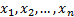comes from a pre-specified population distribution (or a pre-specified family of such distributions).

In its basic form, the test assumes that there are no parameters to be estimated for the distribution being tested, in which case the test and its set of critical values are distribution-free.

However, it is most commonly used where a family of distributions are being tested. For example, we might be testing whether the sample comes from a Normal distribution but without specifying in advance the mean and standard deviation of that distribution. It then becomes necessary to estimate the parameters on which the particular distribution depends and this needs to be taken into account by adjusting the test statistic and/or its critical values.

In its basic form, it involves the following test statistic,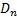, where we are testing the null hypothesis that the data is coming from a distribution with cumulative distribution function (cdf)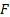: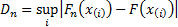where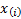is the’th order statistic, i.e. the’th smallest value in the sample,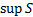is the supremum (i.e. largest value) of the set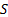and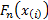is the empirical distribution function, defined in the Wikipedia entry on this test as, in effect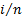, but perhaps more naturally defined as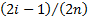, see the Cramer-von-Mises test.

Essentially the same approach can be used when testing whether data comes from a pre-specified family of distributions. However, the statistic must then be compared against critical values appropriate to the family in question and dependent also on the method used for parameter estimation.

The test can also be inverted to give confidence limits on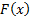itself and a variant can be used to test whether two (or more) underlying one-dimensional distributions differ. Generalising the statistic to more than one dimension is also possible but complicated.